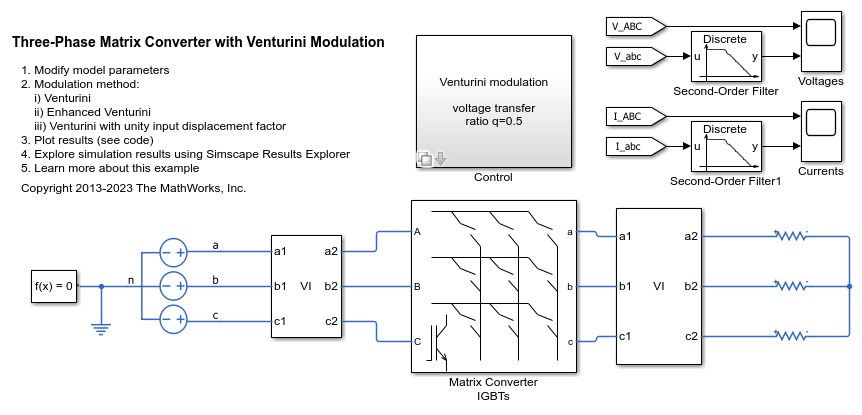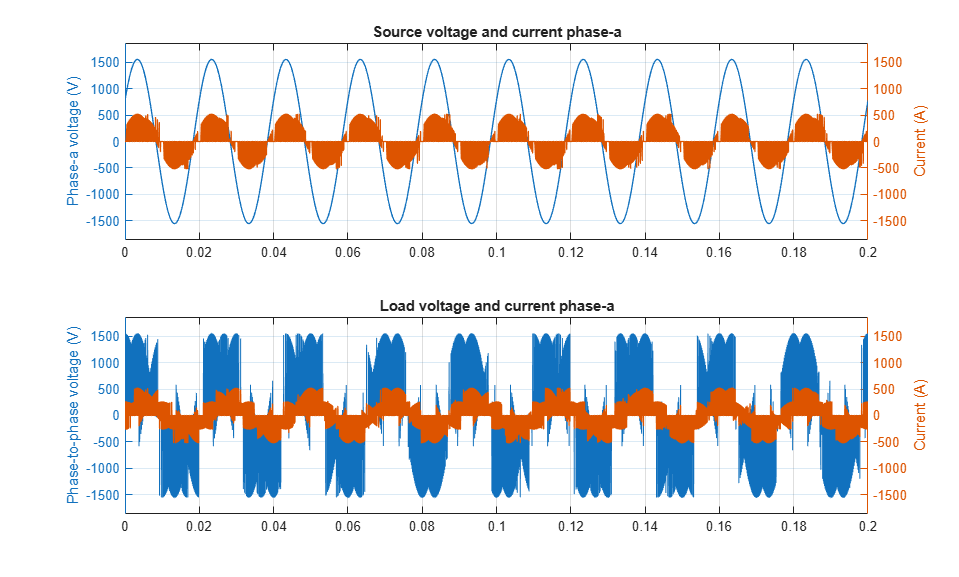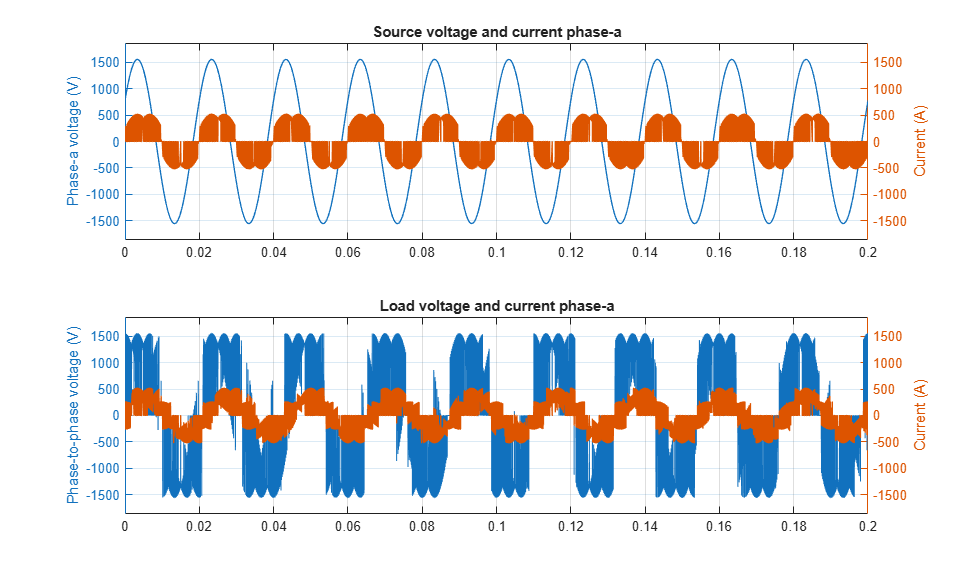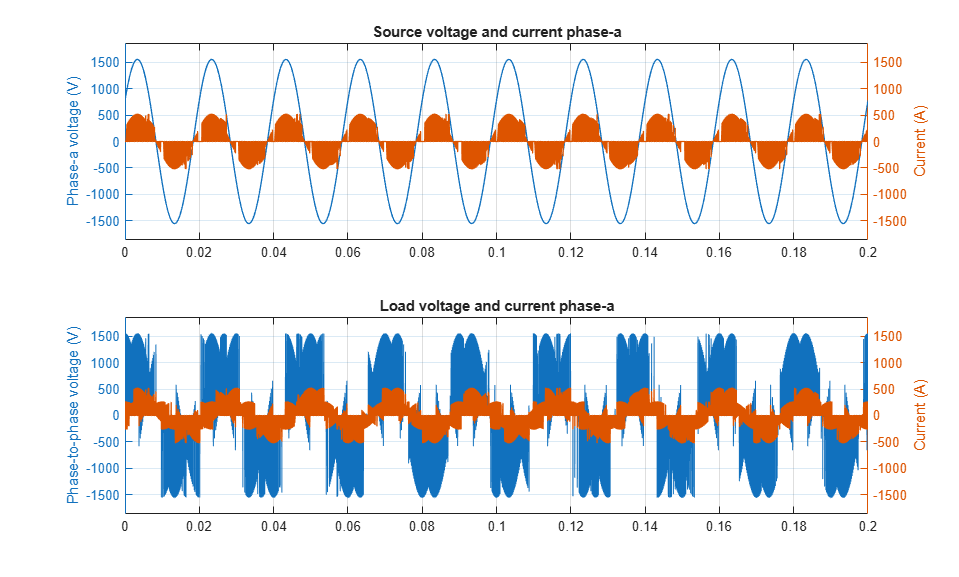# Three-Phase Matrix Converter with Venturini Modulation

This example shows how to use Venturini modulation techniques to compute the duty cycles and logic statements of a three-phase matrix converter that drives a static load. The control subsystem implements three different modulation algorithms: Venturini modulation, third harmonic enhanced Venturini modulation, and third harmonic injection Venturini modulation with unity input displacement factor. The maximum voltage transfer ratio between input and output depends on the modulation technique and it is equal to either q=0.5 or q=0.866. The Scope blocks show the voltages and currents V_ABC, V_abc, I_ABC, and I_abc, where _UPPERCASE is used for inputs and _LOWERCASE for outputs.

### Model### Simulation Results from Simscape Logging

The plot below shows the voltages and currents for the source and load using Venturini method.The plot below shows the voltages and currents for the source and load using enhanced Venturini method.The plot below shows the voltages and currents for the source and load using Venturini method with unity input displacement factor.